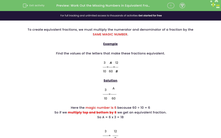# Work Out the Missing Numbers in Equivalent Fractions

In this worksheet, students work out the missing numbers in equivalent fractions.Key stage:  KS 3

Curriculum topic:   Number

Curriculum subtopic:   Use Concepts and Vocabulary for All Numbers

Popular topics:   Numbers worksheets, Equivalent Fractions worksheets

Difficulty level:#### Worksheet Overview

To create equivalent fractions, we must multiply the numerator and denominator of a fraction by the same magic number.

Example

Find the values of the letters that make these fractions equivalent.

 310

 A60

 12B

 310

 A60

Here, the magic number is 6 because 60 ÷ 10 = 6

So, if we multiply the top and bottom by 6 we get an equivalent fraction.

So, A = 6 x 3 = 18

 310

 12B

Here, the magic number is because 12 ÷ 3 = 4

So, if we multiply the top and bottom by 4 we get an equivalent fraction.

So, B = 10 x 4 = 40

 310

 1860

 1240

Let's have a go at some questions now.

### What is EdPlace?

We're your National Curriculum aligned online education content provider helping each child succeed in English, maths and science from year 1 to GCSE. With an EdPlace account you’ll be able to track and measure progress, helping each child achieve their best. We build confidence and attainment by personalising each child’s learning at a level that suits them.

Get started